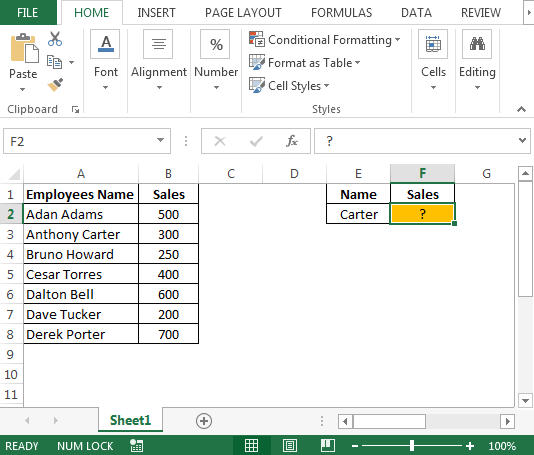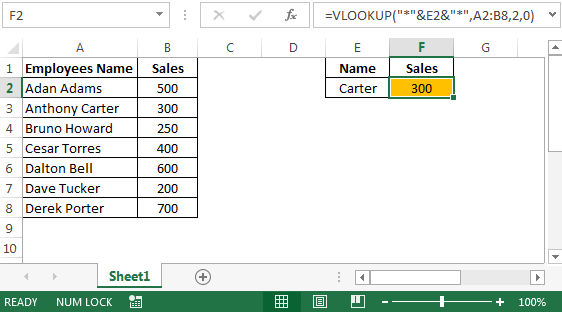# How to Use Wildcard with Vlookup in Microsoft Excel

In case you have not use Vlookup with wildcard then this article is for you & you will really wonder how easy your life would be after you learn this tip. In this article we will learn how to use Vlookup function with wildcard in excel.

Question): I have data that contains first & last name & I want to extract the sales by employees first or last name (not full name). I know how to use Vlookup function but need help on this.

Following is the snapshot of data we have:• We will use Vlookup function to accomplish the output.

Vlookup looks up a value in the selected range of cells in the left-most column & returns the value in the same row in the index-number position.

Syntax =VLOOKUP(lookup_value,table_array,col_index_num,range_lookup)

lookup_value: The value you want to look for

table_array: The table of data contains information from which you want to return the output. Table array should contain at least two columns of data. The first column contains the lookup values. These values can be text, numbers, or logical values.

col_index_num: It contains the data from which you want the information.

range_lookup: Range lookup is a logical value which instructs Vlookup to find an exact or approximate match. The table must be sorted in ascending order. Choices are true or false.

True for Approximate match

False for Exact match

• In cell F2 the formula is
• =VLOOKUP("*"&E2&"*",A2:B8,2,0)• Even if we change the last name from Carter to Anthony the result would be same.

In this way we can use wildcard & Vlookup function together.

1.